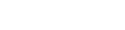• Home
• IPM Aptitude Questio...

# IPM Aptitude Questions Based on Remainders

Author : Palak Khanna

December 23, 2022

SHARE

Attempting IPMAT itself is a challenge and having less time for each paper becomes another. But if you know the right trick, you can manage your time quite easily.

The questions from the remainders concept in the IPMAT maths section are of a certain standard to check your mathematical reasoning. Practising more and more questions from this concept will help you fetch more marks than others.

With the right preparation strategy, students with non-math backgrounds have cleared the exam easily in the past. To ease your preparation, we have compiled the important IPM aptitude questions and answers based on remainders in this post.

So, what are you looking for? Check out the questions from the post below and enhance your preparation.

## What is a Remainder?

In arithmetic, the remainder is defined as the integer left over after dividing one integer by another to produce an integer quotient.

A lot of questions based on the remainder concept are generally asked in the competitive examinations including IPMAT. Therefore, following some IPMAT Maths Preparation Tips and tricks would definitely help you solve these questions in a matter of seconds.

To help you tackle these questions with ease, we have listed important tricks and theorems related to the remainders along with previous year questions and solutions below.

Concept -1

Starting from the basics the general equation for finding remainders is.

Dividend(n) = Divisor(d) x Quotient(q) + Remainder(r)

Let’s understand this concept with the help of an example, if we divide 100/3 we will have:-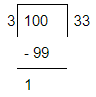Divisor(d) = 3 Dividend(n) = 100

Quotient(q) = 33

Remainder(r) = 1

100 = 3 x 33 + 1Concept 2

If x, y, and z be three random numbers, we know that

1. (x z) gives remainder Rx
2. (y z) gives remainder Ry then find

(i) remainder of (x + y) z

(ii) remainder of (x -y) z

(iii) remainder of (x * y) z

The Trick to solve this question is to replace the unknown number by their respective remainder i.e., (Rx + Ry) / z. The remainder of this term will be the answer now.

Three cases that are possible using this approach are explained below.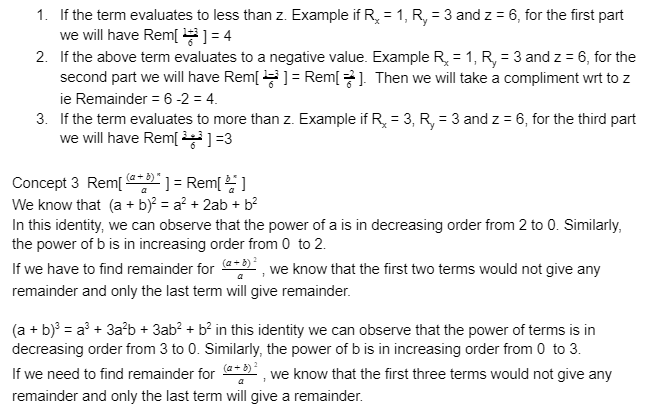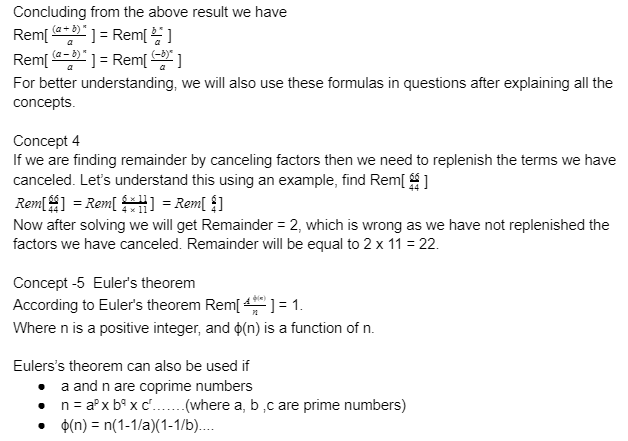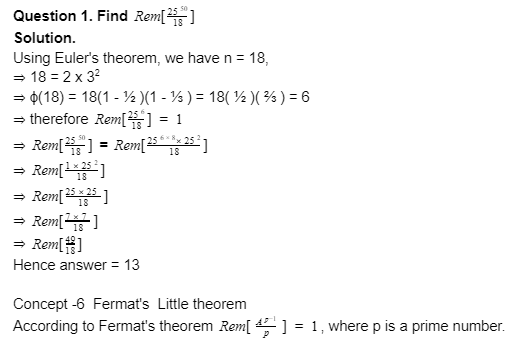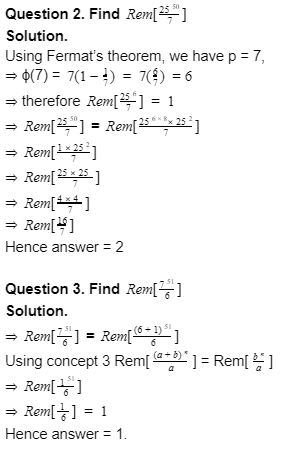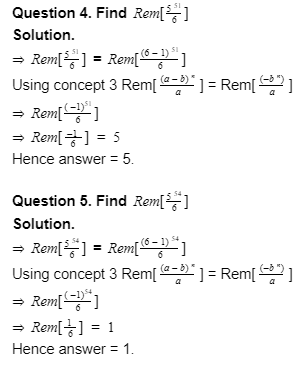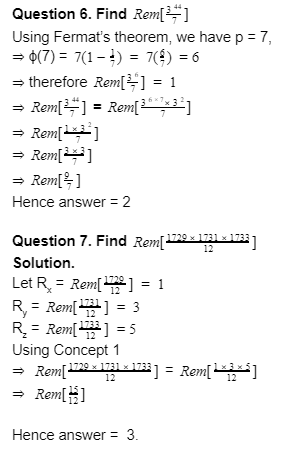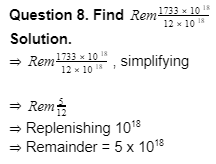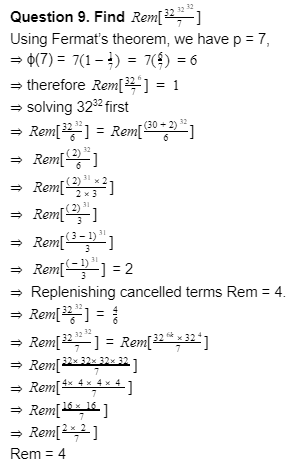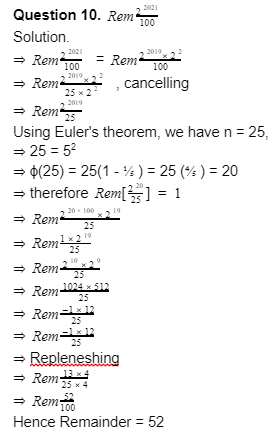### IPM Aptitude Questions & Answers based on Remainders

To help you get an idea about the type of questions asked in the exam, we have provided few sample questions that are curated from the previous year Question Papers for IPMAT.

Question:

In a division problem, the product of quotients and the remainder is 24 while their sum is 10. If the divisor is 5 then the dividend is __________.

Solution :

In this problem, we have given that the product of quotient(q) and the remainder(r) is 24.

i.e q x r = 24 -( i )

And it is also given that their sum is 10

i.e q + r = 10 -( ii )

Divisor(d) = 5.

( i ) and ( ii ) represent the sum and product of a quadratic equation, from ( ii ) putting r = q - 10, in ( i ), we will get q x (10 - q) = 24,

i.e q2-10q + 24 = 0,

Which will give q = 6 or 4.

Therefore one of them will be 6 and the other one will be 4. We do not know yet whether Quotient is 6 and Remainder is 4 or vice versa.

We know that

Dividend(n) = divisor(d) x quotient(q) + remainder(r) .

From the given data, n = 5 x q + r. - ( iii ),

As the divisor is 5 we infer that the remainder obviously will be smaller than 5, therefore the remainder will be 4 and the quotient will be 6. Putting the above-decided value in ( iii ).

n = 5(6) + 4 , therefore n = 34.

Question:

What is the remainder when 123 x 124 x 125 is divided by 9?

Solution :

When 123 is divided by 9, the remainder will be  -3.

While it is -2 when 124 is divided by 9.

Similarly, when 123 is divided by 9, the remainder will be -1

Therefore, final remainder = (-3)(-2)(-1)

= -6

The required positive remainder = 9-6

= 3

Yes. With a proper preparation strategy, it is easy to prepare for the IPMAT Mathematics section.
You can easily improve your speed and accuracy in the IPMAT Exam by solving as many sample papers as possible. Practicing mock tests would definitely improve your time management skills and problem-solving techniques. So, it is advisable to solve mock tests on a daily basis.

Following the expert tips will definitely help you to prepare better for the IPMAT Maths. Begin your preparation by studying from the right books, which cover all the concepts and topics according to the latest exam pattern, and make sure you are aware of the detailed Paper pattern and latest Syllabus of the IPMAT Exam before starting your preparation.

Taking up Mock Tests regularly and solving Sample Papers helps to test your preparation and improve your speed and managing time.

For cracking quantitative ability section, refer to R.D. Sharma or R.S. Aggarwal book. It includes all the concepts from 12th standard explained in depth. It is also easily understandable without any tutor.
It solely depends on you whether you need to take IPMAT coaching or not. If your fundamentals are strong, then it is easy to crack the exam just by solving previous year sample papers and Mock tests. However, if you want to improve your fundamentals then opting for coaching is beneficial.

Some of the general preparation tips to crack the Maths section are: Try to understand the concepts of the topics. Do not go for cramming, Do not try to get into some new topics at the end days of preparation, and Revise the old topics. Go through formulas, and try practicing them. During preparation, check short-cuts too.

• Home
• IPM Aptitude Questio...

# IPM Aptitude Questions Based on Remainders

Author : Palak Khanna

Updated On : December 23, 2022

SHARE

Attempting IPMAT itself is a challenge and having less time for each paper becomes another. But if you know the right trick, you can manage your time quite easily.

The questions from the remainders concept in the IPMAT maths section are of a certain standard to check your mathematical reasoning. Practising more and more questions from this concept will help you fetch more marks than others.

With the right preparation strategy, students with non-math backgrounds have cleared the exam easily in the past. To ease your preparation, we have compiled the important IPM aptitude questions and answers based on remainders in this post.

So, what are you looking for? Check out the questions from the post below and enhance your preparation.

## What is a Remainder?

In arithmetic, the remainder is defined as the integer left over after dividing one integer by another to produce an integer quotient.

A lot of questions based on the remainder concept are generally asked in the competitive examinations including IPMAT. Therefore, following some IPMAT Maths Preparation Tips and tricks would definitely help you solve these questions in a matter of seconds.

To help you tackle these questions with ease, we have listed important tricks and theorems related to the remainders along with previous year questions and solutions below.

Concept -1

Starting from the basics the general equation for finding remainders is.

Dividend(n) = Divisor(d) x Quotient(q) + Remainder(r)

Let’s understand this concept with the help of an example, if we divide 100/3 we will have:-Divisor(d) = 3 Dividend(n) = 100

Quotient(q) = 33

Remainder(r) = 1

100 = 3 x 33 + 1Concept 2

If x, y, and z be three random numbers, we know that

1. (x z) gives remainder Rx
2. (y z) gives remainder Ry then find

(i) remainder of (x + y) z

(ii) remainder of (x -y) z

(iii) remainder of (x * y) z

The Trick to solve this question is to replace the unknown number by their respective remainder i.e., (Rx + Ry) / z. The remainder of this term will be the answer now.

Three cases that are possible using this approach are explained below.### IPM Aptitude Questions & Answers based on Remainders

To help you get an idea about the type of questions asked in the exam, we have provided few sample questions that are curated from the previous year Question Papers for IPMAT.

Question:

In a division problem, the product of quotients and the remainder is 24 while their sum is 10. If the divisor is 5 then the dividend is __________.

Solution :

In this problem, we have given that the product of quotient(q) and the remainder(r) is 24.

i.e q x r = 24 -( i )

And it is also given that their sum is 10

i.e q + r = 10 -( ii )

Divisor(d) = 5.

( i ) and ( ii ) represent the sum and product of a quadratic equation, from ( ii ) putting r = q - 10, in ( i ), we will get q x (10 - q) = 24,

i.e q2-10q + 24 = 0,

Which will give q = 6 or 4.

Therefore one of them will be 6 and the other one will be 4. We do not know yet whether Quotient is 6 and Remainder is 4 or vice versa.

We know that

Dividend(n) = divisor(d) x quotient(q) + remainder(r) .

From the given data, n = 5 x q + r. - ( iii ),

As the divisor is 5 we infer that the remainder obviously will be smaller than 5, therefore the remainder will be 4 and the quotient will be 6. Putting the above-decided value in ( iii ).

n = 5(6) + 4 , therefore n = 34.

Question:

What is the remainder when 123 x 124 x 125 is divided by 9?

Solution :

When 123 is divided by 9, the remainder will be  -3.

While it is -2 when 124 is divided by 9.

Similarly, when 123 is divided by 9, the remainder will be -1

Therefore, final remainder = (-3)(-2)(-1)

= -6

The required positive remainder = 9-6

= 3

Yes. With a proper preparation strategy, it is easy to prepare for the IPMAT Mathematics section.
You can easily improve your speed and accuracy in the IPMAT Exam by solving as many sample papers as possible. Practicing mock tests would definitely improve your time management skills and problem-solving techniques. So, it is advisable to solve mock tests on a daily basis.

Following the expert tips will definitely help you to prepare better for the IPMAT Maths. Begin your preparation by studying from the right books, which cover all the concepts and topics according to the latest exam pattern, and make sure you are aware of the detailed Paper pattern and latest Syllabus of the IPMAT Exam before starting your preparation.

Taking up Mock Tests regularly and solving Sample Papers helps to test your preparation and improve your speed and managing time.

For cracking quantitative ability section, refer to R.D. Sharma or R.S. Aggarwal book. It includes all the concepts from 12th standard explained in depth. It is also easily understandable without any tutor.
It solely depends on you whether you need to take IPMAT coaching or not. If your fundamentals are strong, then it is easy to crack the exam just by solving previous year sample papers and Mock tests. However, if you want to improve your fundamentals then opting for coaching is beneficial.

Some of the general preparation tips to crack the Maths section are: Try to understand the concepts of the topics. Do not go for cramming, Do not try to get into some new topics at the end days of preparation, and Revise the old topics. Go through formulas, and try practicing them. During preparation, check short-cuts too.